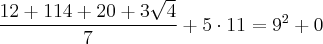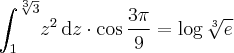A Dozen, a Gross, and a Score,
plus three times the square root of four,
divided by seven,
plus five times eleven,
equals nine squared and not a bit more.

And from there, I found another:Integral z-squared dz
From 1 to the cube root of 3
Times the cosine
Of three pi over 9
Equals log of the cube root of e.

1. Apparently by Leigh Mercer ↩